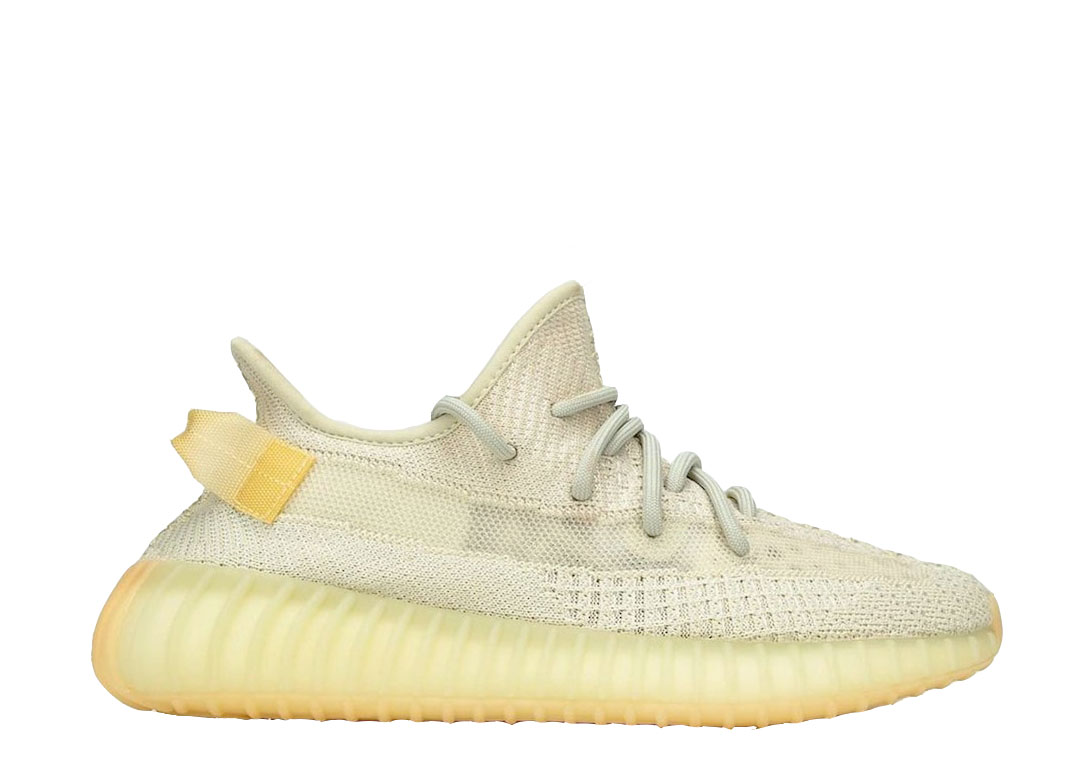Flightkickz the sneaker plug since 2011
US\$

# Yeezy 350 V2 Light UV 2021

US\$ 128.00
US10.5=UK10=EU442/3=28.5 US10=UK9.5=EU44=28CM US11.5=UK11=EU46=29.5CM US11=UK10.5=EU451/3=29CM US12.5=UK12=EU47.3=30.5CM US12=UK11.5=EU46.7=30CM US4.5=UK4=EU36.7=22.5CM US4=UK3.5=EU36=22CM US5.5=EUR38=UK5=23.5CM US5=UK4.5=EU37.5=23CM US6.5=UK6=EU39=24.5CM US6=UK5.5=EU38.5=24CM US7.5=UK7=EU402/3=25.5CM US7=UK6.5=EU40=25CM US8.5=UK8=EU42=26.5CM US8=UK7.5=EU411/3=26CM US9.5=UK9=EU431/3=27.5CM US9=UK8.5=EU422/3=27CM
Flightkickz

• Detail

It’s understandable that fans of Kanye West and adidas (XETRA:ADS.DE +1.19%) ‘ YEEZY BOOST 350 V2 may think they’ve seen it all. Since the second iteration of West and the Three Stripes’ YEEZY BOOST 350 made its retail debut back in September 2016, we’ve seen dozens of colorways with everything from zebra stripes to reflective detailing, exoskeleton-like upper cages and gum soles. However, the adidas YEEZY line is in a constant state of reinvention — and the 350 V2 has appeared in an unparalleled new style dubbed “Light.”

CopyYeezy 350 V2 Light UV 2021
color:red,size:xxl
Subtotal: US\$ 0
View My Cart

Company Info